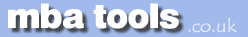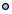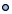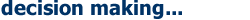NavigationMarketingFinanceOperationsEconomicsStatisticsStrategyMeetingsToolboxCommunicationmbatools.co.ukcompiled by

gari jenkinsDecision Tree Analysis: choosing between options by projecting likely outcomes.

How to use tool:
Decision Trees are excellent tools for helping you to choose between several courses of action. They provide a highly effective structure within which you can lay out options and investigate the possible outcomes of choosing those options. They also help you to form a balanced picture of the risks and rewards associated with each possible course of action.

Drawing a Decision Tree
You start a Decision Tree with a decision that you need to make. Draw a small square to represent this towards the left of a large piece of paper. From this box draw out lines towards the right for each possible solution, and write that solution along the line. Keep the lines apart as far as possible so that you can expand your thoughts.

At the end of each line, consider the results. If the result of taking that decision is uncertain, draw a small circle. If the result is another decision that you need to make, draw another square. Squares represent decisions, and circles represent uncertain outcomes. Write the decision or factor above the square or circle. If you have completed the solution at the end of the line, just leave it blank.

Starting from the new decision squares on your diagram, draw out lines representing the options that you could select. From the circles draw lines representing possible outcomes. Again make a brief note on the line saying what it means. Keep on doing this until you have drawn out as many of the possible outcomes and decisions as you can see leading on from the original decisions.

An example of the sort of thing you will end up with is shown in Figure 1:Once you have done this, review your tree diagram. Challenge each square and circle to see if there are any solutions or outcomes you have not considered. If there are, draw them in. If necessary, redraft your tree if parts of it are too congested or untidy. You should now have a good understanding of the range of possible outcomes of your decisions.

Evaluating Your Decision Tree
Now you are ready to evaluate the decision tree. This is where you can work out which option has the greatest worth to you. Start by assigning a cash value or score to each possible outcome - how much you think it would be worth to you if that outcome came about.

Next look at each circle (representing an uncertainty point) and estimate the probability of each outcome. If you use percentages, the total must come to 100% at each circle. If you use fractions, these must add up to 1. If you have data on past events you may be able to make rigorous estimates of the probabilities. Otherwise write down your best guess.

This will give you a tree like the one shown in Figure 2:Calculating Tree Values
Once you have worked out the value of the outcomes, and have assessed the probability of the outcomes of uncertainty, it is time to start calculating the values that will help you make your decision.

Start on the right hand side of the decision tree, and work back towards the left. As you complete a set of calculations on a node (decision square or uncertainty circle), all you need to do is to record the result. You can ignore all the calculations that lead to that result from then on.

Calculating The Value of Uncertain Outcome Nodes
Where you are calculating the value of uncertain outcomes (circles on the diagram), do this by multiplying the value of the outcomes by their probability. The total for that node of the tree is the total of these values.

In the example in Figure 2, the value for 'new product, thorough development' is:

0.4 (probability good outcome) x £500,000 (value) = £200,000
0.4 (probability moderate outcome) x £25,000 (value) = £10,000
0.2 (probability poor outcome) x £1,000 (value) = £200
+ £210,200

Figure 3 shows the calculation of uncertain outcome nodes:Note that the values calculated for each node are shown in the boxes.

Calculating The Value of Decision Nodes
When you are evaluating a decision node, write down the cost of each option along each decision line. Then subtract the cost from the outcome value that you have already calculated. This will give you a value that represents the benefit of that decision.
Note that amounts already spent do not count for this analysis - these are 'sunk costs' and (despite emotional counter-arguments) should not be factored into the decision. When you have calculated these decision benefits, choose the option that has the largest benefit, and take that as the decision made. This is the value of that decision node.

Figure 4 shows this calculation of decision nodes in our example:In this example, the benefit we previously calculated for 'new product, thorough development' was £210,000. We estimate the future cost of this approach as £75,000. This gives a net benefit of £135,000.
The net benefit of 'new product, rapid development' was £15,700. On this branch we therefore choose the most valuable option, 'new product, thorough development', and allocate this value to the decision node.

Result
By applying this technique we can see that the best option is to develop a new product. It is worth much more to us to take our time and get the product right, than to rush the product to market. It is better just to improve our existing products than to botch a new product, even though it costs us less.

Key points:
Decision trees provide an effective method of decision-making because they:

• clearly lay out the problem so that all options can be challenged

• allow us to analyse fully the possible consequences of a decision

• provide a framework to quantify the values of outcomes and the probabilities of achieving them

• help us to make the best decisions on the basis of existing information and best guesses.

As with all decision-making methods, decision tree analysis should be used in conjunction with common sense - decision trees are just one important part of your decision-making tool kit.Toolbox @ Complexity @ Decision Making - PMI- RACI @ Creativity